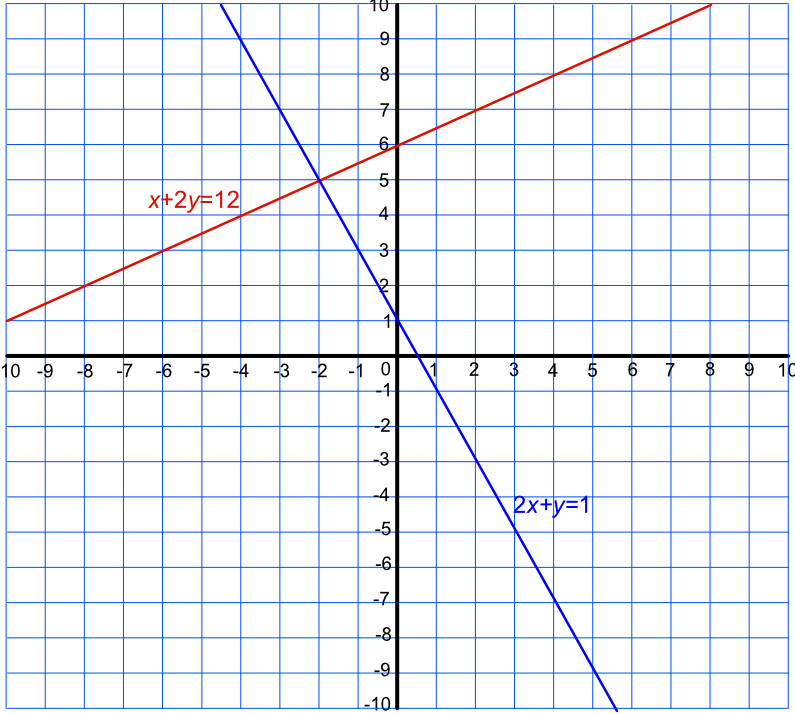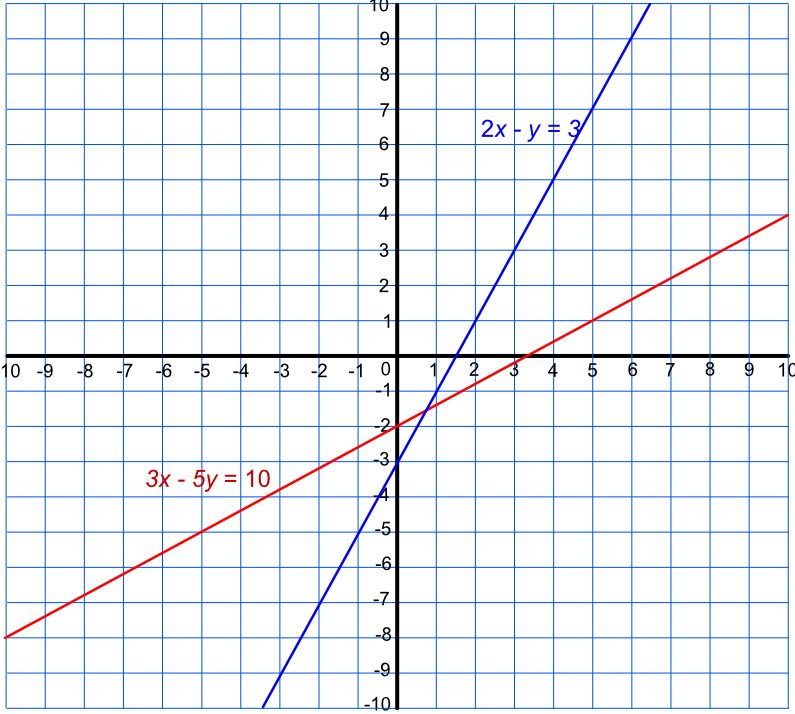Simultaneous Equations using a Graph

# Simultaneous Equations using a Graph

GCSE(F), GCSE(H),

Approximate solutions for simultaneous equations can be found by plotting a graph of the two functions and determining the point at which they cross. The coordinates of that point give the solution to the two equations:

• Arrange the equations so that both equations have the same unknown on one side;

• Plot both functions;

• Identify the crossing point of the functions;

• Check accuracy by substituting the values into the original equations.

The solutions are the intersection points, on a graph, of the linear and quadratic functions. There may be 0 (no intersection points), 1 (the graphs touch) or 2 (the graphs cross) intersection points.

## Examples

1. Find an approximate solution to the simultaneous equations 2x+y=1 text( and ) -x+2y=12 by plotting a graph.

Answer: x=-2 text( and ) y=5

Rearrange both equations: 2x+y=1 -> y=1-2x and -x+2y=12 -> y=0.5x+6 and plot:Obtain the intersection point for the solution (-2, 5)

Check the answer: 2(-2)+5=1 (true) and -(-2)+2(5)=12 (true).

2. Find an approximate solution, using a graph, to the simultaneous equations 2x-y=3 text( and ) 3x-5y=10.

Answer: x=0.7 and y=-1.6

Rearrange both equations: 2x-y=3 -> y=2x-3 text( and ) 3x-5y=10 -> y=0.6x-2 and plot.Obtain the intersection point for the solution (0.7, -1.6)

Check the answer: 2(0.7)-(-1.6)=3 (good) and 3(0.7) - 5(-1.6)=10.1 (approximately correct).